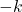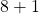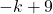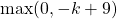# ILP Part 70 — Elements reordering

This is the seventieth part of the ILP series. For your convenience you can find other parts in the table of contents in Part 1 – Boolean algebra

Today we are solving the following problem: we have a set of elements with ranks (higher rank is better) and want to reorder them to maximize the revenue. However, some elements are pinned to positions and can be moved at most one slot away. Only eight top elements are considered for the cost function (they fit in the UI element), also, elements closer to the beginning are worth more.

Sounds simple:

We start with ranks and pinned positions. We create variables and make sure positions are different. Next, we add ranges for each element and pin elements if possible.

Finally, the goal. We use the simple if condition: if an element is in first 8 slots then its rank is multiplied by the position with simple linear scaling (first element is worth eight times more than the eighth element), otherwise the item doesn’t change the goal function.

Output:

So it took 1,5 minutes to find the solution. Can we do better?

Hard part here is we use the condition which can be represented in ILP but is pretty heavy operation. However, we can use simple math to make it faster:

We take elementin position. We first negate the position and get, we then increase it byso we getand then take the maximum. Notice what happens: if the element was in first 8 positions then this value is transformed into proper ordering (first element is worth eight times more than the eighth element). Otherwise we get zero. We then multiply the value by the element’s rank.

Output:

Notice we get some rounding errors but at the end of the day it’s the same solution calculation in 40 seconds (almost twice as fast as previous approach). Can we do better?

Instead of having general integer variables let’s use binaries:

We use two dimensional array of binaries, first dimension is element number, second dimension is element position. Binary variable indicates whether i-th element can be assigned to j-th position.

First we block positions for pinned elements. Next, we make sure there is one element in given position. Finally, we make sure element is in one position only.

For goal we define calculations much easier: we iterate over premium positions and can precaculate the factor. We then multiply it by the binary variable. Result:

Looks like manual “variable unrolling” is way better. Generally, avoid using comparisons and prefer binary flags (especially if you can precalculate them outside of the ILP model).